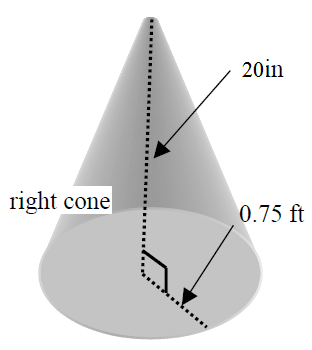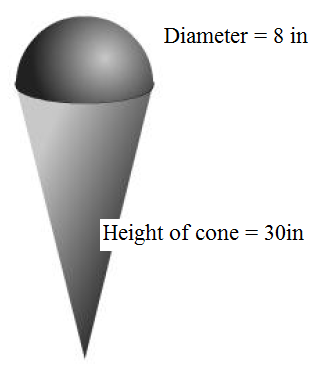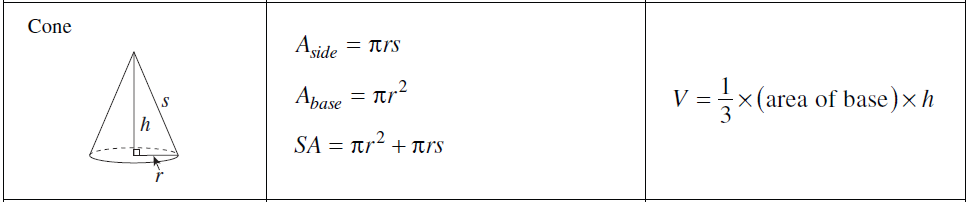# Surface area and volume of cones

0/0
0/2
##### Examples
###### Lessons
1. Find the surface area and the volume of the following cones:

1.2.###### Free to Join!
StudyPug is a learning help platform covering math and science from grade 4 all the way to second year university. Our video tutorials, unlimited practice problems, and step-by-step explanations provide you or your child with all the help you need to master concepts. On top of that, it's fun - with achievements, customizable avatars, and awards to keep you motivated.
• #### Easily See Your ProgressWe track the progress you've made on a topic so you know what you've done. From the course view you can easily see what topics have what and the progress you've made on them. Fill the rings to completely master that section or mouse over the icon to see more details.
• #### Make Use of Our Learning Aids###### Practice Accuracy

See how well your practice sessions are going over time.

Stay on track with our daily recommendations.

• #### Earn Achievements as You LearnMake the most of your time as you use StudyPug to help you achieve your goals. Earn fun little badges the more you watch, practice, and use our service.
• #### Create and Customize Your AvatarPlay with our fun little avatar builder to create and customize your own avatar on StudyPug. Choose your face, eye colour, hair colour and style, and background. Unlock more options the more you use StudyPug.
###### Topic Notes
We just learned how to calculate the surface area and volume of pyramids and cylinders in the previous lessons. Now, we will look at cones - objects that have circle bases like cylinders and pointy tops like pyramids. Just like pyramids and cylinders, there are formulas for surface area and volume of cones. We will also try out some questions on composite solids that consist of cones too.

## What is a cone?

Before we deal with calculations for the surface area and volume of cones, let's understand what a cone is first.

A cone is one of many geometric shapes. It has a flat surface on one of its ends and it comes to a point on the other end. The cones that we will be discussing in this section are right circular cones. What exactly is that? It's a cone that has a circle on its flat end, and a point that is 90 degrees from the center of the circle.

As we will be dealing a circle, be prepared to use pi and radius in our calculations. Take a moment to review this if you need before proceeding.

## How to find the surface area of a cone

The surface area of a cone calculates the area surrounding the cone, and the surface of the circular base. We can easily find it with the following formula:

$Surface\;area = \pi r^2+ \pi rs$

How would this work in a math problem?

Question:

Find the surface area of the following cone.

Solution:

Surface area of cone

$Surface\;area = \pi r^2+ \pi rs$

From the graph, we know the height (h) = 20in, radius (r) = 0.75ft. We need to find the slant (s) to calculate the surface area using the Pythagorean Theorem. But in order for us to do proper calculations, we'll have to convert the (h) and (s) into the same unit (inches) first. You can notice that one of the measurements is in feet, and one of them is in inches. To convert it, you'll get the following:

$0.75ft = 9m$

So we have height (h) = 20in, radius (r) = 9in. The slant is actually the hypotenuse of the right angle inside the cone. To find its length, we can now apply the Pythagorean Theorem:

$S=\sqrt{9^2+20^2}$

$S\approx 21.9in$

Lastly, we can plug in the numbers into the formula:

$SA= \pi (9)^2+ \pi (9)(21.9)$

$SA\approx 875in^2$

The cone surface area is roughly $875in^2$

## How to find the volume of a cone

So how do we find the volume of a cone? The cone volume is a measurement of how much space there is inside a cone. There is a volume of a cone formula to help you find this:

$Volume= \frac{1}{3} \times (area\;of\;base) \times h$

Let's try it out on an example. It's the same cone as the previous walkthrough, so you should be quite familiar with it already.

Question

Find the volume of the following cone:

Surface area of cone

Solution

Volume of cone

$Volume= \frac{1}{3} \times (area\;of\;base) \times h$

First, we need to calculate the area of the cone's base

$Area\;of\;base=\pi r^2$

$Area\;of\;base=\pi (9)^2$

Now, we can plug apply the numbers into the formula for volume of a cone

$Volume= \frac{1}{3} \times (\pi (9)^2) \times (20)$

$Volume \approx 1696.5 in^3$